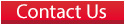Products Products Overview Monthly Deals Estimate Savings (ROI) MHI Financing Airtorch™ Process Heaters Superheated Steam Generators e-Ion Plasma™ Microheaters™ Heating Elements Furnaces Robust Radiator™ Thermoplate™ HotTop™ Control Panels Gas Panels Data Acquisition System Shop Online General Information About MHI About MHI Our Mission Scholarly References Leave Feedback Advantages Economics Testimonials Career Opportunities Useful Links Commercial Steam Cleaner Muffle Furnace Electric Steam Boiler High Temperature Furnace Steam Boiler Temperature Converter Enter a number& click on the "Calculate" button °F= °C

# MHI Watt Calculator

## MHI 101: Energy, Power and Thermodynamics Tutorial

Engineering Units for Energy and Power

The JOULE (J) is a commonly used unit of energy.  The BTU is also an unit of energy.
Energy units employed by electric companies are the kWhr (Kilo-Watt-Hour for electric) or Therm (for gas).

1000J = 1kJ (KiloJoule) = 0.9485 BTU=0.0002778 kWhr

1 kWhr = 0.03413 Therm [U.S.]

One Therm (TH) = 100,000 BTU

1PJ (PetaJoule)= 1,000,000,000,000,000  i.e. 10^15 Joule.

1EJ (ExaJoule) =1,000,000,000,000,000,000 i.e. 10^18 Joule.

The Watt (W) is a unit for power i.e. the energy used (or supplied) per unit time.

1 J/s (Joule per second) = 1 W (Watt)

Power is sometimes expressed in alternate units like BTU/hr or Horsepower (hp)

Power-Units Conversion 1kW (KiloWatt) = 3414 BTU/hr

0.746kW= 1hp

Power rating of steam-boliers is in units of BHP (boiler -horsepower)

One BHP (Boiler Power)= 33,500 BTU/hr = 9.803 kilowatts

(The Boiler-BHP power unit is a bit confusing becasue BHP could be confused with brake-horsepower (bhp) which is an auto-industry terminology)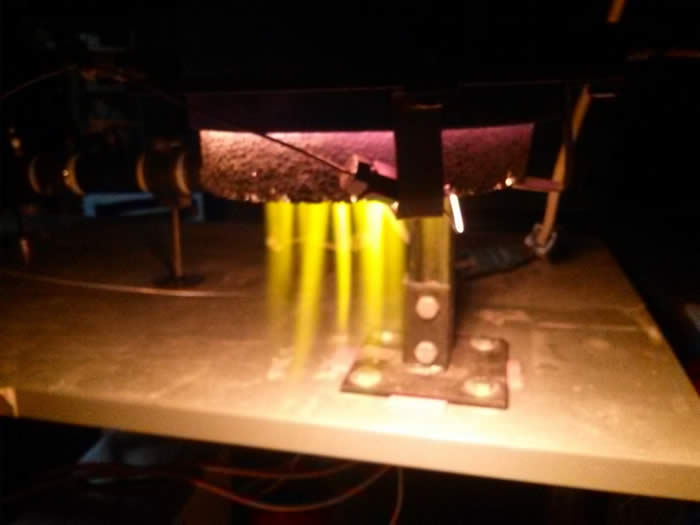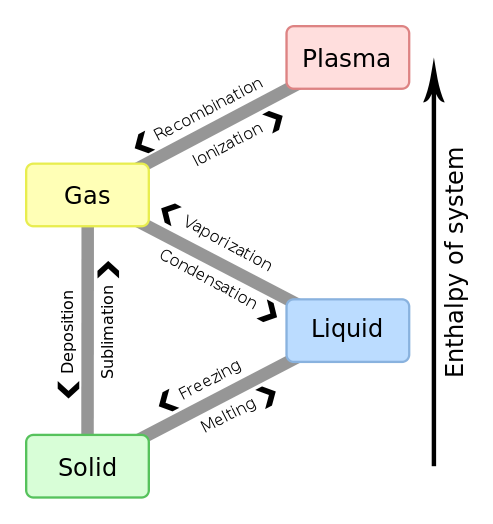What is Energy - What is Power?

Energy:  We often encounter power and energy in our daily lives. The concept of Energy (or more appropriately the concept of the exchange of Energy) is related to the latent ability to perform a task called work, transfer mass or transfer heat.  The task is always to cause a change i.e. enable an objective.

Objective: A change in the volume (or shape), chemistry, temperature or density of an object of interest is a possible objective.

What happens to energy when it is employed for an objective? Energy degrades when energy is used for an objective. However the amount of energy is unchanges.

Units: Energy is commonly measured in Joules (J).  In the Physics literature, natural units are used for energy, namely the Planck energy unit (Ep) which is equal to 1.956 x 10^9 J (about 2 Billion Joules). The estimate is that we use more than a hundred-billion Planck units of energy every year.  In other units we can say that on a gloabal scale, humans use about more than 500EJ's per year (1EJ = ~0.947 Quad).  1EJ (ExaJoule) = 10^18 Joule.  Most objects* have a mass and energy associated with them. Energy exchanges for carrying out an objective for a process can occur by the transfer of Work, Heat or Mass, between a system and its surroundings or vice-versaThere are many measurable properties such as like P (Pressure), V (volume) and T (temperature) which also are used for defining energy.   The energy of an object is both internal (microscopic), like the vibrational energy of molecules or external (macroscopic) energy like kinetic or potential energy.  Often the external energy is called mechanical energy and sometimes also PV energy.  The internal energy is given the symbol U.  The total energy (internal plus mechanical) is given the symbol E

*We use objects and cotrol volumes as terms that are interchangeble in this tutorial.  Objects can be of a phase called solid, liquid gas, plasma or in a supercritical condition or a two or more phase mixture.

Energy is conserved - Ywa this is the First-law of Thermodynamics (theconservation of energy is a consequence of Emmy Noether's theorem on symmetry). But what does it mean when we want to consever energy? When used the energy is conseved but the quality degrades.   The main reason for energy conservation stems from the desire to conserve the quality of energy (not the quantity).

The amount of Energy (used/exchanged) is a relative quantity.  Only the change in energy is measurable. This is because an energy exchange is referenced always to an arbitrary base-state of your choice.  Base-states are arbitrary, e.g. the base-state could be the room-temperature and/or room-pressure such as 0K, 25°K, 72°For 278K or the melting point of a known pure substance.    Regardless of the base-state, the change-amount will be the same for the energy exchanged because energy is conserved.

What is Power:   Power is the amount of energy that is converted in one unit of time (e.g. in a second). Devices are rated by their power. Power is oomph! A more powerful device will be able to convert the same amount of energy faster (speedier) than a less powerful device.  Generally speaking, power is the rate at which you can do something, e.g. higher power can make an automobile accelerate faster, make a reaction go faster, and so on.  A Watt (W) is a unit of power.   1 J/s (Joule per second) = 1 W (Watt).  Because the Watt is a small unit power of a device is rated in kW or BTU/hr.  1kW (KiloWatt) = 3414 BTU/hr.  Brake horsepower (bhp) is the measure of an engine's horsepower before the loss in power caused by the gearbox and drive train.  Electric cars and Hybrids often have a dash-display for kW.  Note that 1hp (horse power)=0.746kW.

The JOULE (J) is the most commonly used unit of energy but is so small that KiloJoules (kJ) is the more commonly used engineering-unit for energy.  The BTU is also an unit of energy.   Yet another unit is KWhr (Kilo-Watt-Hour).  1kJ (KiloJoule) = 0.9485 BTU=0.0002778 kWhr.   Almost all electric is measured in units of kWhr of energy.   This is is called one unit of electric.  Almost all gas energy is measured in Therms or Million BTU's (MBTU).

As these are all units of energy they can be converted from one unit to another. One Million BTU (MBTU)= 10.002 Therm [US].   1 Therm [U.S.] = 29.30 kilowatt hour  (1 kilowatt hour = 0.03413 therm [U.S.]).   Often using electric heating is less polluting at the use point.  Additionally, electric heaters can be efficiently/accurately controlled.  For natural gas heating about 117 pounds of CO2 are produced for one million BTU (293 KWh) of energy produced by combustion.  When purchasing gasoline, diesel or oil for combustion, the price is still approximately the same for one unit of energy (about 5-14 US cents/kWh) depending on the geographical location because of a variation in gasoline taxes.   Do not expect major differences in the real price of one unit of energy when employed for a similar performing power-car whether electric or gas.  One should note that when things move at the speed of light (e.g. photons)  they have no mass but plenty of energy!  More about this in the thinking-quiz questions below but this speed in not relevant for us in this tutorial.  Energy is commonly measured in Joules (J).  In Physics, natural units are used for energy reporting, namely the Planck energy unit (Ep) which is equal to 1.956 x 10^9 J (about 2 Billion Joules). The estimate is that we use more than a billion Planck units of energy every year.

Why should we care to conserve energy even though by definition it is a conserved quantity?  Because conserving energy is not the same as conserving the quality of energy.  More below.... section about entropy.

Energy Price For historical reasons we use many different units for power and energy. The commonly used units are given above.  For more units, the calculator(s) below will help you to convert energy, or power from one type of units to another.  You can do more with the calculator.  Say you wanted to get a feel for the amount of energy used in terms of equivalent amount of gasoline burnt, or in terms of how much ice the same energy would melt; you can do that also!  Have fun!  Energy and Power have cost and prices associated with them  (i.e. they are traded in some way).  Joules and Watts are SI units (ice the scientific measurement units that most countries accept).  SI stands for Le Système International d'unités.As mentioned above the current prices of electric and gas energies are listed by utility companies e.g. Both KWhr and Therm are units of energy which your electric company may use.    Electricity (electric energy) is normally priced for every KWhr used, while gas (fuel energy) is priced by the number of Therms used.  1kJ (KiloJoule) = 0.9485 BTU=0.0002778 kWhr (kilowatt hour).   One Million BTU (MBTU)= 10.002 Therm [US].   10 Therm [U.S.] = 293.0 kilowatt hour.   When checking prices you will note that the energy delivered to you whether electric or gas will cost you about the same per unit of energy!

Smart power industrial devices are also available today.  Similar to SmartPhones, the SmartSteam and SmartPlasma devices employ a lot less power than previously possible for the same objective.   These new devices also provide an enhanced productivity compared to devices of the past.

Price of Energy: Approximately About 9 US cents/kWhr.

Price of Power Consuming Devices?  Cars, refrigerators and motors are often priced by the device horsepower (power); light bulbs are often priced by their output watts (power); natural gas is sold by its combustible energy content namely therms (energy) and of course the supply-demand situation of the gas; electricity by kilowatt-hours (energy). More powerful devices cost more but you may recoup the cost as it may do the same objective is lesser time this savings in wasted energy and hours used.  Convective units are normally priced by power (examples are Convection Air Movers and Air Conditioners); Furnaces are priced by kilowatts (power) and their temperature capability in Kelvin (K); and air conditioners by tons or BTU's per hour (power).  Power multiplied by the time of use of that power, gives you energy.  The SI units for energy is Joules. Expect to pay a little more when demanding higher power! or expect to earn more when delivering products or service with a high-power high-productivity device.   When you purchase a energy conversion device (say electrical energy to heat or steam), the device will often be rated by its "power".  This enables you to calculate how much energy you will use (and pay for) by simply multiplying the rated power by the hours used.  When using electric power the commonly used units of energy are kilowatt-hours or kWhrs (which is an unit for energy,  1kWhr= 3600,000J).   Each electric unit that you pay for, is normally one kilowatt-hour (also sometimes called one unit).  When you pay for units, your US electric utility bill contains a price the for the units used during normal usage, and the price for units used in a "demand penalty" condition (peak hour use).  You should always carefully watch this penalty number, and contact your electric utility provider to find ways for reducing the "penalty" charge. Efficient devices use lesser energy and allow on off control in order to optimize energy payment., Devices like the OAB(R) and Airtorch™ are highly power transfer efficient.   The typical order of magnitude of power-rating range (kW) for commonly used devices is shown in the table below. The range also reflects new devices that consume less power compared to older ones.  The order of price paid in the US for power consuming devices is also shown.  The lower kW devices when they perform the same objective in lesser time than the higher power devices are called smart power devices.  Smart power devices tend to be energy efficient, offer low foot-print/weight and increase productivity.

 Order of magnitude of Power consumed by devices (Power Rating) Light Bulbs Home Refrigerators/Washer/Dryers Cars kW 0.03 - 0.2 2 - 5 20 - 300 Price (order of magnitude only) in US dollars ~ \$1 ~ \$1000 ~ \$13000 - \$100000

Thermal Sciences and Thermodynamics.  A tutorial.

Energy and Power are the key concepts that are taught in a subject called Thermodynamics.  The science of Thermodynamics also deals with issues like energy transfer and other variables that define the direction of any process.  Einstein considered thermodynamics to be the most important discipline because it is a basis for understanding why time change occurs in only one direction.   Thermodynamics also enables you to understand if something is at equilibrium (equilibrium generally indicates unchanging measurable properties).  At the microscopic level (think atoms and smaller entities that make up atoms) however things are always moving.  Thermodynamic considerations at the microscopic level are only possible by taking into account an aggregate of small things (like a mole of molecules ~ 6.02x 10^23 atoms).  This branch of thermodynamics is called Statistical Thermodynamics.  At the macroscopic level we can define measurable properties like pressure (P) and temperature (T) when an object or control volume (V) (of a shape that describes the object of study) is moving at a fixed velocity or is stationary.  The study of thermodynamics at the macroscopic level level is called Classical Thermodynamics.  The connection between the two branches was described by Boltzmann and Claussius who were able to describe and interrelate macroscopic properties by describing the microstates available to the system for distributing its energy. At high enough temperatures the only part of statistical mechanics that we need to know is called Maxwell-Boltzmann statistics where a distribution of energy across microstates/particles is Gaussion in nature.   Thermodynamic is a subject that is used in an important manner across many different scales of understanding the world from large power generation plants to the shapes of the internal structure of matter.  The key laws of Thermodynamics that are discussed below are applicable to small scale understanding where quantum mechanics is important and also to larger scale understanding where classical Newtonian mecahnics is valid.  This is a good place to note that the price of energy production for our daily activities is very much related to energy innovationA Short Article about the future directions in the science of Thermodynamics.

The Joule (J) is the unit of energy in SI units.  Other common units are BTU's, kJ (kiloJoules), and kWhr (kiloWatt-hour) or units from groupings of properties such as PV, TS or uM, where P is absolute pressure, V is Volume, T is absolute temperature, S is entropy (explained below in detail),  u is chemical potential (per unit mass) and M is mass.  The term PV is the only one which contains a grouping of commonly measurable properties.  We also define Enthaly (H) as equal to U+PV. When solving energy related problems it is common to define a system. Here U is the internal energy and P and V are volume and pressure respectively.   The system of interest may be imagined to be enveloped in what is termed the control volume boundaries and the system itself is referred to as the control volume.  This control volume is the object of study in many energy related problems. In the paragraphs below you will learn that that the efficiency of any machine is limited by the First Law of Thermodynamics and sometimes by the Second Law of ThermodynamicsThe first law conserves energy whilst allowing changes in the form of energy, whereas the second law deals with reordering the type of energy and any change in the ability of the energy to prerform an objective.   For solving energy related problems inside a system of interest, the use of a control volume concept is particularly important to use (particularly when the system is a gas).  A system could also denote a fixed condensed-matter (solid or liquid) volume, if it is largely incompressible.  The condensed matter volume is then substituted for control volume when solving problems.  The boundaries of a control volume are expected to be very thin with respect to other lenght scales of the energy related problem. Sometimes several phases are involved in a problem for example, when considering the melting boiling or a human heart (or lipid membrances of a cell) or a gas turbine engine.  For such systems of study a particular unchanging phase boundary defines most of the closed boundary between the system and its surrounding universe.  Four states of mattter within a control volume are common to study, namely, solid, liquid, gas and plasma. Here is an approximate diagram of how these phases may transform into the other as a function of temperature or enthalpy change.

There are many measurable properties such as like P (Pressure), V (volume) and T (temperature).  These and other properties define the thermodynamic state of any system. A system may be associated with properties that are not directly measurable.   Energy and Entropy are two of them.  Energy, E (Joules) is always conserved (such a conservation process is known as the first law of thermodynamics).  The Entropy, S (loosely defined as a property when changed, modifies of the quality of the energy in a given material or control volume) is not conserved.  This is not a difficult concept when you realize that some properties like mass are conserved and some like density are not conserved.  Of course we are only speaking about properties of materials which are at rest or moving at much slower than the speed of light (although one must note here that photons are not conserved).  Weird science happens when moving at the speed of light. New words like antimatter make their way into our vocabulary when studying phenomena that involves the speed of light e.g. matter and antimatter can transform to energy and vice-versa!  Something can appear from nothing- seemingly!

Entropy is not a difficult concept.  It is a thermodynamic variable (property of a material at equilibrium) just like any other property.  The overall entropy of the Universe is not conserved, so when a process occurs- the state of the system is changed by the process - and new entropy may be generated and distributed in the system or surrounding.  You will learn about other properties called Enthalpy, Landau Potential, Helmholtz Free Energy and Gibbs Free Energy in MHI 102.  Finally in MHI 103 you will learn about entropy generation, Sgen.  Suffice it to know for now that these variables reflect or modify the amount of useful work that can be recovered from any system while holding other select thermodynamic properties especially Energy and Temperature, constant for a system that changes its state.  Entropy is associated with the order prevalent in a system or object.   More order - lesser entropy. More disorder - higher entropy, when compared at the same temperature or for the same material.  An easy way to appreciate this is to think about a system containing a known material, say water, at the melting point at one-atmosphere pressure.   If at the melting point the water is in the form of a solid it has lesser entropy than if the same amount of water when in a liquid form, at the same temperature (melting point).   When you posses energy with the lowest entropy, you posses the most useful form of energy for conversion to work, i.e. should work be your objective and only when you are comparing for constant energy.  However the inherent value of the energy (quality) to you depends on what you need it for.  For example, if you are cold and you need heat, your objective is not for an ordered form of energy that takes you (allows you e.g. to drive) to another cold place, but more for an increase in temperature which is best gained by heat transfer to you.  Similarly many chemical processes require heat for faster kinetics (i.e. for improved productivity).  On the other hand when you have an automobile, it seems important that you be able to order the energy of the automobile motion in order to stay in your defined lane!

A note about temperature:  The Centigrade temperature scale was developed by Anders Celsius (1701–1744) by separating the melting point (~0°C) and boiling point of water (~100°C) by 100 degrees.  Daniel Gabriel Fahrenheit (1686–1736) developed the Fahrenheit scale, also by assuming that 100 degrees separated the coldest and hottest temperatures in a year yet understanding but pegging the fixed points on the scale to a thermodynamic invariant temperature, ice-water-ammonium chloride equilibrium (0°F), ice-water equilibrium (32°F), and body temperature (~98°F).  The mercury-thermometer was mainly invented by an experimental-mathematician, Ole Christensen Romer (1644-1710) with Fahrenheit’s input.  Romer was also the first to quantitatively measure the speed of light, introduce standardized street-lighting and improve nautical navigation with high precision instruments. Today we use thermocouples, thermistors and semiconducting materials with a known band-gap for the accurate measurement of temperature. We also define the lowest possible temperature (0° K) with a Kelvin (or SI) temperature-scale named after William Thompson Kelvin. We also use Rankine, Romer, Newton, Delisle and Reaumur temperature-scale(s) for temperature measurement? And also a Planck temperature-scale that ranges from 0-1 (Tp) where 0 (Tp) = 0(°K) and 1 (Tp) = 1.416×10^32 (°K).

The inherent energy (E) of a system is useful to know because it can achieve an objective (e. g. heating or lighting or locomotion).  When this energy is exchanged by the system with its surrounding (a system can strictly speaking also choose to rearrange the way it partitions energy within itself but we will leave this to MHI 102) a certain process path is taken.  When the State of Energy of a system changes, the path taken for this change is important to know because it relates to the objective of the intended change.

Entropy generation rate is fundamental to shape and path selection.  Chaos or Order are simply different types of objectives for a process.  A process involves a starting state and the final state (the objective).  The  objective may be a higher temperature (more chaotically energy arrangement) or a lower temperature (less chaotic energy arrangement).  Sometimes Chaos is better than Order and sometimes the other way- depends on the objective. When a change of state occurs there are possible implications on the work ability from the new state.    The chances are high that you may have during a process one may have converted some of the mechanical energy to a more disordered form of energy (by processes such as friction) or rearranged the microscopic distribution of energy - these are called irreversiblities.  Much more on this in the laws of thermodynamics section below. Sometimes the objective of changing a state involves the choice of a determined process path.  In an ideal situation, when process work is the intended objective, one seeks a process that does not cause any irreversibility, and consequently no entropy is generated (see bulleted points below).  Electrochemical processes, and processes where energy is converted to other forms of energy without any generation of entropy (work to work) are close to such ideal (reversible) processes.  But almost no processes is really reversible.  Modern process designs are those where the process otimization involves using what was in the past thought of as waste.  If during an irreversible entropy generating process, some heat is produced, this heat can be a useful feature also.  Such new concepts are used to make electric cogeneration power plants. In thermodynamic terms, any gradient (i.e. the difference of a thermodynamic property with distance) in potential, including gradients in temperature, composition, height or charge can cause energy to flow spontaneously with a corresponding generation of entropy.   Whenever entropy is generated, the process is called irreversible.  Note that the word irreversible also has the connotations of the direction of time and is neither inherently good or bad -  remember it depends on your objective.  Both time and energy flow "spontaneously" only in a certain direction.  This is the direction that produces positive new entropy or none at all.  In the module, MHI 103, you will learn about a clever way that natural processes choose when trying to spontaneously find a process-path.  Nature's clever way for process-path and shape selection is called the MEPR principle. MEPR (sometimes simply called MEP) stands for 'maximum entropy production rate'.   New articles review this in the context of the History of Thermodynamics and why the future belongs to new plasma processes like the cascade e-Ion.

A system may changes its energy content when it interacts with the surroundings.  A process-path that defines the exchange process for energy exchange.  This path represents a change in the thermodynamic-property(s) variable during which energy is exchanged by the system with its surroundings.  The exchange of energy however occurs by either heat transfer, work (both boundary and non boundary) or by mass transfer.  Heat and Work are only recognized during the process path.  They are not thermodynamic properties because we cannot associate them with a state of a system at equilibrium.  Heat flow, on account of energy transfer occurs from a difference in temperature.

Heat flows from a higher to lower temperature only.  Heat flow can be further classified as conduction, convection or radiation.  Heat flow is designated by the symbol q (Watts/m2).  For radiation q is proportions to T4.  For convection q is proportional to T and for conduction heat flow is also proportional to the T, where T is some average absolute temperature between the high and low temperatures between which the heat is transferred spontaneously.   When you convert energy from one form to another, you may create new entropy (almost always) - and when you do that, the process is called irreversible (because you generate new entropy and end-up degrading the quality of energy).   Process-paths need not be continuous or reversible and not just because of quantum effects! (more in MHI 103).  Let us define P and V as our symbols for pressure and volume respectively.  We can now define a new useful property, Enthalpy (H)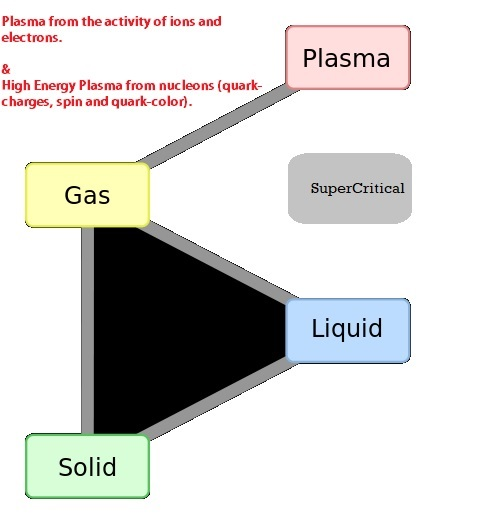Electron and Nuclean Plasma and Other Phases of Matter

(defined as H= E+PV).  Enthalpy is an important thermodynamic property which is particularly useful to know during phase change processes.  When enthalpy is changed (say by combustion or heat transfer) of a control volume, the process for the change is called irreversible, because entropy is generated by the process.   Efficiencies depend on your objective. Using the best energy efficient device is important - you end up degrading or wasting the least amount of energy for your objective.  Efficiency therefore generally depends on how much of energy gets converted to the objective.  However, Energy is always conserved and there is a best efficiency when converting heat to work which is a limiting law of nature (described more below- see section on Laws of Thermodynamics).

What is Energy Efficiency (First Law and Second Law Efficiencies)

Rule: There is no process that can violate the First or Second Law of Thermodynamics. Each law provides a limitation on the objective during any process.

The first law states that energy is always conserved (but of course may be converted from one form to other form by heat flow, mass flow or work interactions). In this context, the efficiency indicates the efficiency of your conversion to the form of energy you require from the form of energy you start with. For example you may wish to tap the electric energy (also called electric work) from an electric power socket and wish to convert it to the thermal energy contained in a hot gas -for example with an with an Airtorch™. The time averaged efficiency of this process will be defined by the power delivered into or by by the hot gas when compared to the power input from the electric source. The First law efficiency depends on the objective of the energy conversion/use and can be as high as 100%.

The second law efficiency is more complicated. When examining a process for second law limited process conversion, the efficiency is the measure how close the process is when compared to a reversible process (see above for the meaning of reversible). It is important to know at this stage that the second law can be stated in many forms e.g., the Kelvin-Planck Statement or the Clausius Statement, and has to do with the impossibility of spontaneously converting disordered forms of energy totally into ordered forms of energy without doing extra work (the penalty). Simply stated one is limited from making all heat into work. This law sets the ultimate limits on your car efficiency (assuming that the car has a heat producing combustion engine) or solar cell efficiency.

The limitation imposed by the First Law is that energy cannot be created or destroyed and for all purposes energy is a conserved quantity.   Energy efficiency can be 100% when referred to the first law if there are no unintended losses during the energy exchange (conversion-use).

The limitation imposed by the Second Law is that entropy can only be created i.e. new entropy can only be generated - once created, entropy can never be destroyed. This is why there is a direction to heat flow i.e. only spontaneously from hot to cold (if heat could flow from cold to hot, then one will destroy some entropy of the Universe which is not allowed by the second law).  Energy efficiency when referred to the the second law is maximum only when no new entropy is produced egg a totally frictionless process or a process in which there are no temperature, concentration or pressure gradients.  By implication, if the first law for any process does not indicate a 100% efficiency for a stated energy-transfer objective, then almost always the second law cannot indicate a maximum second law efficiency for the same process.

Entropy is not a conserved quantity (unlike energy which is always conserved). Any exchange of energy of the system and surroundings can produce new entropy (called irreversible exchange) or keep it the same (called reversible exchange). One should not confuse new entropy generation with entropy (S) as a thermodynamic property (one of the properties of a condensed matter material or control volume at equilibrium). The second law limitation only applies to entropy generation, not to the change of entropy between the states of a system (a system can have a higher or lower entropy depending on how it interacted with the surroundings during a process). Regardless of the process path is chosen, the entropy generation can only be zero (a reversible path) or greater than zero (a irreversible path). The higher the temperature of the source when the sink is maintained at room temperature, the higher will be the efficiency of the conversion of thermal energy into an ordered form of energy (say mechanical energy). There is no significant penalty going the other way i.e. from work to heat ice an ordered form of energy can be converted to a disordered form- theoretically without penalty.

What is exergy? Is it efficiency?

The Third and also a Zeroth Law of Thermodynamics. The Third law states that reaching a zero temperature in Kelvin is not possible with a finite number of steps (it is also stated as inferring that at 0K the entropy is zero or for a perfect crystal at 0 K there is no translation, rotation or vibration of molecules.  The zeroth law of thermodynamics states that when comparing systems in equilibrium with a standard reservoir they will be in equilibrium with each other if they are in equilibrium with the reservoir.  But the concept of equilibrium is not yet important to grasp as we will learn more about it in MHI 102.  For now it will suffice to know that equal temperature, pressure or chemical potential (so that no mass is exchanged) provides equilibrium within and across a control volume.  Given below are some properties of entropy.  Not all laws of thermodynamics are seemingly known yet!  How to photons and magnons produce entropy?

•Entropy will increase as we increase the temperature of the perfect crystal.  Higher temperature - more entropy.
•Molecules gain vibrational motion and the degrees of freedom (related to entropy) increase with an increase in the temperature.
•As we heat a substance from absolute zero, the entropy must increase.
•The entropy changes dramatically when there is phase-change. When a solid melts, the molecules and atoms have a large increase in freedom of movement.  Boiling generally implies a much greater change in entropy than melting.  One can understand this because the solid to liquid involves only a few bonds broken whereas a gas has very weak bonds between gas atoms or molecules.  An ideal gas is defined as one that has no bonds between individual atom or molecules species.  An ideal gas exhibits an equation of state (one that connects temperature pressure and temperature) which is well known to us, namely PV=NRT.  Here R is the universal gas constant R=8.314 J/mol.K (0.0831 bar dm3 mol-1 K-1 Note that we had defined H(enthalpy)=U+PV.  Because U can be measured from any arbitrary state for an ideal gas, the enthalpy and internal energy of an ideal gas are only a function of temperature by definition.  N is the number of atoms or molecules (in moles) in our control volume.  Later on we will also encounter an ideal liquid, which is defined as one where the concentrations are eaqual to a property known as "activity" (let us leave this issue to the MHI 102 module).  Before getting to this module lets us first enjoy some fun facts including the issues with ionization.  The two diagrams below should be very understandable in this regard.  In MHI 102 and 103 we are going to study ionic and other reactions!  Given below (bulleted) are some typical efficiencies encountered in everyday processes.  Some limitations are from the first law limitations and some from the second law limitations.  Which one matters to you is dependent on the specific objective that you seek.

Figures that explain ionization (partial credit Wikipedia https://en.wikipedia.org/wiki/Fermionic_condensate#/media/File:Phase_change_-_en.svg) are shown below.. We have already discussed how enthalpy, entropy and temperature all increase when one of them is increased for a substance that remains the same (same chemical species) during the increase or a phase change.  Ionization is required for plasma formation and here we start changing to multi species like ions and electrons.  We will deal with this in MHI 102..  The cascade e-ion does for example produces a plasma..  As we learn more about chemical potentials we will understand this concept with more accuracy in due course.

• Burning fossil fuel to get useable heat - about 85% (running a gas-fired water heater, or making steam to power a turbine...some heat goes up the smokestack).   Here we are speaking about first law efficiency.
• Burning fossil fuel to get electricity - about 33% (active world-wide research area to improve several types of efficiencies).  The big drop in efficiency comes from the second law limitation which states that not all heat (a disordered form of energy) can be converted to work (an ordered form of energy).   Here we are speaking about the second law efficiency.

• Sunlight to electricity in a PV (photovoltaic) cell - about 10% (active world-wide research area to improve conversion efficiency, some polymer photovoltaic's are now reporting over 35-44% i.e. close to silicon technology).  Can you tell if this process has a second law limitation? Hint - consider if any heat is produced.  Also see answer to quiz problem 6 below.

• Storing electrical energy in a battery (charging it) and pulling it back out -about 90%

• Converting electrical energy into mechanical energy with an electric motor - about 85%

• Fuel Cell about 60% or lower.  Theoretically this can be even as high as about 92%.   Electrochemical processes are limited in efficiency by how much enthalpy of the electrochemical reactions can be used as free energy and produce useful work (called exergy).  (Any entropy generation that occurs from the of mixing of constituents limits the electrochemical conversion efficiency).

• IC (internal Combustion) engine about ~ 30% (second law thermal efficiency).  Higher temperatures are better for efficiency.

• Wind energy about 59.5%.  Wind energy mills convert the kinetic energy of the wind to rotational shaft energy which can be used to create electrical energy.   The limitation of wind energy conversion limitation comes from having to cater for residual kinetic energy in the downwind past the windmill blades.  Here the first and second laws are both in play. Frictional heat causes entropy generation as do eddys.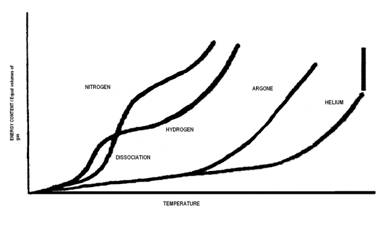Our Featured Product Brands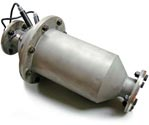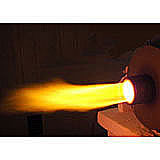Furnaces up to 1800°C Airtorch™ Gas Heaters up to 1200°C Superheated Steam up to 1300°C Microheaters up to 1900°C Electric Flame up to 1900°C

The Internet's Top Rated Watt and Energy Calculator - Created By MHI

Energy

(used or delivered)

Time

(This is the time over which the energy was used or delivered)

Joules

Watts (UNIT OF POWER)
Watt Hours Kilowatts (UNIT OF POWER)
Kilowatt Hours Horsepower (UNIT OF POWER)
BTU BTU/hr. (UNIT OF POWER)
Therms tons (ODD LOOKING UNIT BUT USED FOR POWER ALSO)
This is the average power in full sunlight falling on a square foot surface, directed toward the sun, and collecting hours each day.
(To Clear Results Above)

** The following table illustrates how various energy storage systems would store this amount of energy **

Chemical Mechanical (Kinetic) gallons of gasoline ( pounds) pounds of natural gas ( cubic feet at psi), pounds of hydrogen Gas ( cubic feet at psi), a lead-acid battery weighing pounds a high-tech flywheel weighing about pounds a pound car traveling at miles per hour melting a block of ice weighing pounds heating a gallon tank of water by degrees F. evaporating pounds (about gallons) of water heating a pound hunk of concrete by degrees. We are the Leader in Our Industry and strive to reach New Milestones in all of Our Products - Allowing our New and Loyal Customers to transform their Great Inventions into Successful Innovations.    (To Clear Results Above)

Note that unless a phase change is indicated, the table below does not consider latent heat.

 To convert power from the unit in the first column to the unit in the second, multiply by the conversion factor in the third column. Example : 1 Btu/hr = (1 x 0.0003929) Horsepower = 0.0003929 Horsepower
 To Convert To Multiply By Btu/hr Horsepower 0.0003929 Btu/hr Gram-cal/sec 0.07 Btu/hr Foot-pounds/sec 0.2162 Btu/hr Watts 0.2931 Btu/min Kilowatts 0.01757 Btu/min Horsepower 0.02356 Btu/min Foot-lbs/sec 12.96 Btu/min Watts 17.57 Foot-pounds/min Kilowatts 0.0000226 Foot-pounds/min Horsepower 0.0000303 Foot-pounds/min Kg-calories/min 0.000324 Foot-pounds/min Btu/min 0.001286 Foot-pounds/min Foot-pounds/sec 0.01667 Foot-pounds/sec Horsepower 0.000818 Foot-pounds/sec Kilowatts 0.001356 Foot-pounds/sec Btu/min 0.07717 Foot-pounds/sec Kg-calories/min 1.01945 Foot-pounds/sec Btu/hr 4.6263 Horsepower Kilowatts 0.7457 Horsepower Kg-calories/min 10.68 Horsepower Btu/min 42.44 Horsepower Foot-lbs/sec 550 Horsepower Watts 745.7 Horsepower Foot-lbs/min 33000 Horsepower (boiler) Kilowatts 9.803 Horsepower (boiler) Btu/hr 33479 Horsepower (metric) Horsepower 0.9863 Kilowatts Horsepower 1.341 Kilowatts Kg-calories/min 14.34 Kilowatts Btu/min 56.92 Kilowatts Foot-lbs/sec 737.6 Kilowatts Watts 1000 Kilowatts Foot-lbs/min 44260 Watt (international) Watt (absolute) 1.0002 Watts Kilowatts 0.001 Watts Horsepower 0.001341 Watts Horsepower (metric) 0.00136 Watts Kg-calories/min 0.01433 Watts Btu/min 0.05688 Watts Foot-lbs/sec 0.7378 Watts Btu/hr 3.4129 Watts Foot-lbs/min 44.27 Watts Erg/sec 107 Watts (absolute) Btu (mean)/min 0.056884 Watts (absolute) Joules/sec 1
 MHI Product Quick Links  -    Robust Radiator™   |     Airtorch™    |     Hybrid Furnaces™    |     Inline Radiator™   |     Flat Heaters™     Thermoplate™    |     Specialty Furnaces Fluidized Bed    |     Plasma    |     HotTop™    |     New Surface Heaters for Electronic Applications    |     Fuel Cell Applications

1. Q1 : What kind of energy is converted by our digestive system.  How efficient is our digestive system compared to other living beings?  Answer
2. Q2: A closed system is one in which no mass crosses the boundaries of your system.  An isolated system is one in which no mass and no energy crosses the boundary.  What is an open system?  What is a steady state system?  Is a steady state (time invariant) energy conversion process reversible?  Answer
3. Q3: What if the device in Q2 was isentropic? Adiabatic?  Answer
4. Q4: Friction produces heat, and heat flow produces entropy. I understand this.  But I have read that entropy is generated when two or more substances are mixed?  Why? Answer
5. Q5: Is there any theoretical limitation to wind energy conversion efficiency? Answer
6. Q6: Is there any theoretical limitation to solid-state solar energy conversion efficiency? Answer

One Therm (TH) = 100,000 BTU
One cubic foot of home gas = 1,000 BTU (approximately)
One MCF (1,000 cubic feet) = 10 Therms (approximately)
One cubic foot of Butane gas = 3,200 BTU
One gallon No. 6 fuel oil = 149,000 BTU
One gallon propane = 92,000 BTU

Price of Energy:  The price of energy depends on the source but is not that different between different forms of commonly available energy (e.g. gas, gasoline or electric).  Price comparisons for the same amount of energy are given by utility companies for example here.  In the US, when comparing gas and electric an approximate rule of thumb could be that gas energy is about 75% the price for the same quantity of electric energy.   However electric machines are generally much more efficient than gas heaters.  For example, the OAB or Cascade e-ion can save you even 90% of traditional energy use in relevant applications.  These new machines can dramatically influence operational costs.  Often using electric heating is less polluting at the use point.  Additionally, electric heaters can be efficiently/accurately controlled.  For natural gas heating about 117 pounds of CO2 are produced for one million BTU (293 Kwh) of energy produced by combustion.

Volume

1.0 cubic foot = 7.48 gallons = 28.27 liters
0.134 cubic foot = 1.0 gallons = 3.78 liters

Continue to MHI 102   Radition Thermodynamics.

MHI 103 Questions and Answers to Ponder

MHI 104 Chemical Kinetics

 All calculations above and in the conversion tables are approximate only, and should not be used for detailed engineering calculation that may require a high degree of precision.  Please check all values against more established calculators and published books.  None of the values above are guaranteed to be accurate.

Links to Apply for Energy Efficiency Grants - MHI-Inc

Contact MHI to design the best solution for your application.

All Trademarks and Trade symbols on this website unless otherwise identified belong to Micropyretics Heaters International Inc.

I

©Micropyretics Heaters International Inc. 1995 - 2023
750 Redna Terrace Cincinnati, OH 45215, USA
Telephone: 513-772-0404 | Fax: 513-672-3333